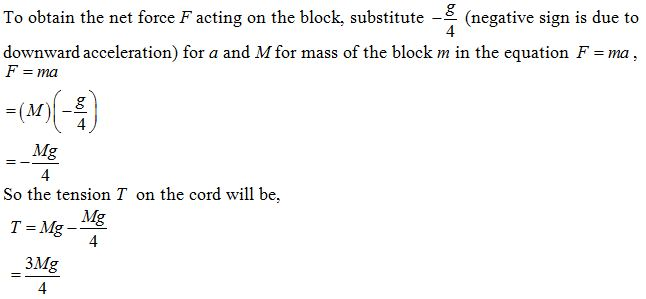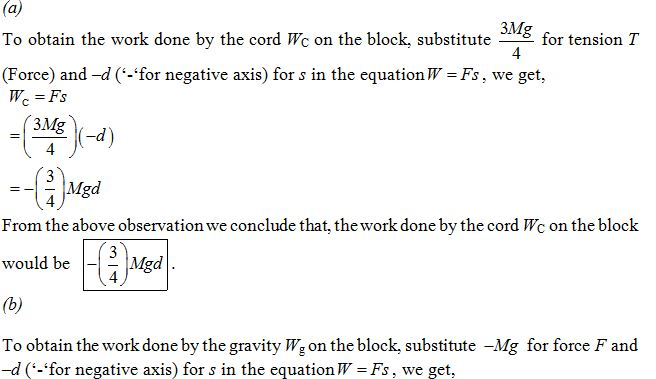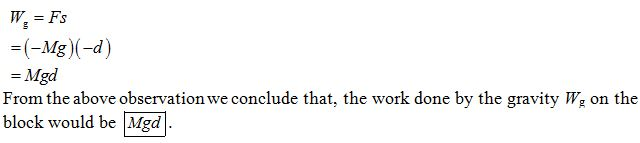×#### Thank you for registering.

One of our academic counsellors will contact you within 1 working day.

Click to Chat

1800-1023-196

+91-120-4616500

CART 0

• 0

MY CART (5)

Use Coupon: CART20 and get 20% off on all online Study Material

ITEM
DETAILS
MRP
DISCOUNT
FINAL PRICE
Total Price: Rs.

There are no items in this cart.
Continue Shopping
```
A cord is used to lower vertically a block of mass M a distance d at a constant downward acceleration of g/4. (a) Find the work done by the cord on the block. (b) Find the work done by the force of gravity.

```
5 years agoNavjyot Kalra
654 Points
``````
5 years ago
```							Check the net force on the object and then write the equation of motion.Tension and gravity are working on the object and motion is in direction of gravity. Hence the equation of motion is,Mg-T= ma.   -(1)As the acceleration of the object is given as he as the acceleration of the object is given as G divided g divided by 4.T=3mg/4(A) work done =force×displacementAs we know that displacement is in opposite direction of force.Hence workdone is (-ve).W=-f.dW=-(3/4)×Mg×d(B) now work done by gravity is in direction of displacement hence it  is positive.W=force(of gravity)×displacement W=Mgd
```
3 years ago610 Points
```							Dear student,Please find the solution to your problem. We have,T – Mg = MaT = Mg + M( – g/4)   = ¾ MgHence, work done by tension, WT = T.(–d) = – Td                                                  = – ¾ Mgd The work done by Gravity, Wg = Fg.(d)                                            = Mgd Thanks and regards,Kushagra
```
5 months ago
Think You Can Provide A Better Answer ?

## Other Related Questions on Mechanics

View all Questions »### Course Features

• 101 Video Lectures
• Revision Notes
• Previous Year Papers
• Mind Map
• Study Planner
• NCERT Solutions
• Discussion Forum
• Test paper with Video Solution### Course Features

• 110 Video Lectures
• Revision Notes
• Test paper with Video Solution
• Mind Map
• Study Planner
• NCERT Solutions
• Discussion Forum
• Previous Year Exam Questions